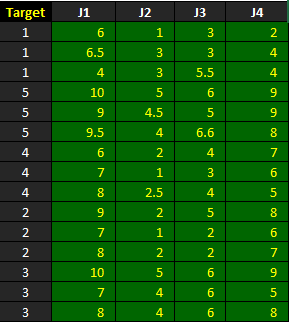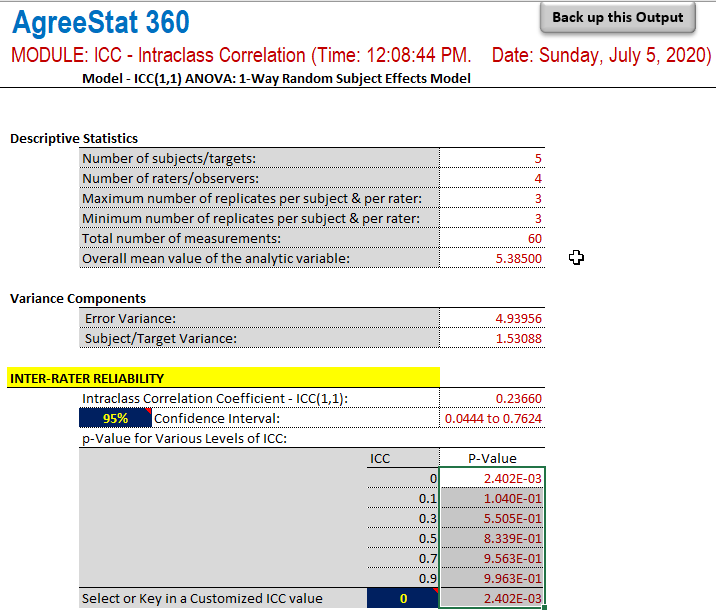﻿ AgreeStat/360: intraclass correlation coefficients for 3 raters or more under the one-way random subject effects model

#AgreeStat AnalyticsResearch & Software for Analyzing Inter-Rater Reliability Data

## Intraclass Correlation Coefficients for 3 Raters 1-Way Random Subject Effects Model

Input Data

The dataset shown below is included in one of the example worksheets of AgreeStat 360 and can therefore be downloaded from the program (This video shows how you may import your own data to AgreeStat360). It contains the ratings that 4 judges J1, J2, J3, and J4 assigned to 5 subjects.

The objective is to use AgreeStat360 to compute the intraclass correlation coefficient and associated precision measures under the 1-way random subject effects model.Analysis with AgreeStat/360

To see how AgreeStat360 processes this dataset to produce the intraclass correlation coefficients and associated precision measures, please play the video below.  This video can also be watched on youtube.com for more clarity if needed.

Results

The output that AgreeStat360 produces is shown below and has 3 parts. The basic descriptive statistics constitute the first part.  The second part shows the variance components, while the third part shows the inter-rater reliability estimate along with its precision measures. No intra-rater reliability can be calculated under the current model.

Each of the 3 parts of this output contains statistics separately for each of the 3 analytic variables vglobal, vlad, and vlcx.

• Descriptive statistics: The first part of this output displays basic statistics about the number of non-missing observations as well as their mean values or number subjects.

• Variance components: This section will typically show the error and subject variances. These variance components are used for calculating the inter-rater reliability coefficient, and could help explain its magnitude.

• Inter-rater reliability: This part shows the inter-rater reliability coefficient, its confidence intervals and associated p-values.  If needed, you can modify both the confidence level and the ICC null value to update the corresponding confidence intervals and p-values.